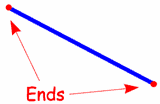# Compare Points, Lines, Line Segments, And Rays

Approved & Edited by ProProfs Editorial Team
At ProProfs Quizzes, our dedicated in-house team of experts takes pride in their work. With a sharp eye for detail, they meticulously review each quiz. This ensures that every quiz, taken by over 100 million users, meets our standards of accuracy, clarity, and engagement.
| Written by Jodenedevan
J
Jodenedevan
Community Contributor
Quizzes Created: 1 | Total Attempts: 1,781
Questions: 10 | Attempts: 1,781SettingsThis quiz will find out what you know about points, lines, line segments, and rays.

• 1.

### What is this?

• A.

A point

• B.

A line

• C.

Line segment

• D.

Ray

C. Line segment
Explanation
The word "segment" is important, because a line normally extends in both directions without end.

Rate this question:

• 2.

### What is this?

• A.

a point

• B.

A line

• C.

Line segment

• D.

Ray

B. A line
Explanation
A line has no ends! If it does have ends it would be called a "Line Segment".

Rate this question:

• 3.

### A_______ is a line with a start point but no end point (it goes to infinity)

• A.

A line

• B.

A ray

• C.

A line segment

• D.

A point

B. A ray
Explanation
If it has just one end it is called a "Ray"

Rate this question:

• 4.

### ___________ is an exact location. It has no size, only position. ?

• A.

A line

• B.

A line segment

• C.

A point

• D.

A ray

C. A point
Explanation
A point is an exact location in space that has no size or dimensions. It represents a specific position and does not have any length, width, or height. Unlike other options like a line, line segment, or ray, which have length and extend infinitely in one or both directions, a point is simply a single location in space.

Rate this question:

• 5.

### A straight line that goes on forever in one direction?

• A.

A point

• B.

A line segment

• C.

A ray

• D.

A line

C. A ray
Explanation
A ray is a straight line that extends infinitely in one direction from a specific point. It has a starting point but no ending point. This is different from a line segment, which has two distinct endpoints. Therefore, a ray is the correct answer for a straight line that goes on forever in one direction.

Rate this question:

• 6.

### A straight line that goes on forever in two directions?

• A.

A line

• B.

A point

• C.

A line segment

• D.

A ray

A. A line
Explanation
A line is a straight path that extends infinitely in both directions. It has no endpoints and continues indefinitely. Unlike a line segment or a ray, a line does not have a specific starting or ending point. It can be thought of as a never-ending series of points that are all in a straight line.

Rate this question:

• 7.

### A part of a line that has two endpoints?

• A.

A point

• B.

A line

• C.

A line segment

• D.

A ray

C. A line segment
Explanation
A line segment is a part of a line that has two distinct endpoints. It is a straight path between two points and does not extend indefinitely in either direction. Unlike a line, which continues infinitely in both directions, a line segment has a definite length and can be measured. Therefore, a line segment is the correct answer for this question.

Rate this question:

• 8.

### A __________ is a location in space.

• A.

A line segment

• B.

A ray

• C.

A point

• D.

A line

C. A point
Explanation
A point is a location in space that has no size or dimension. It is represented by a dot and is used to indicate a specific position or coordinate in a geometric figure or system. Unlike other options like a line segment, ray, or line, a point does not have any length, width, or direction. It is simply a single position in space, making it the correct answer for this question.

Rate this question:

• 9.

### What is this?

• A.

A point

• B.

A line

• C.

A ray

• D.

A line segment

D. A line segment
Explanation
A line segment is a straight path between two points that has a definite length. It is different from a line because it has a specific starting and ending point. In this case, the given image is showing a straight path with two distinct endpoints, indicating that it represents a line segment.

Rate this question:

• 10.

### What are these called?

• A.

Lines

• B.

Points

• C.

Line segments

• D.

RaysBack to top